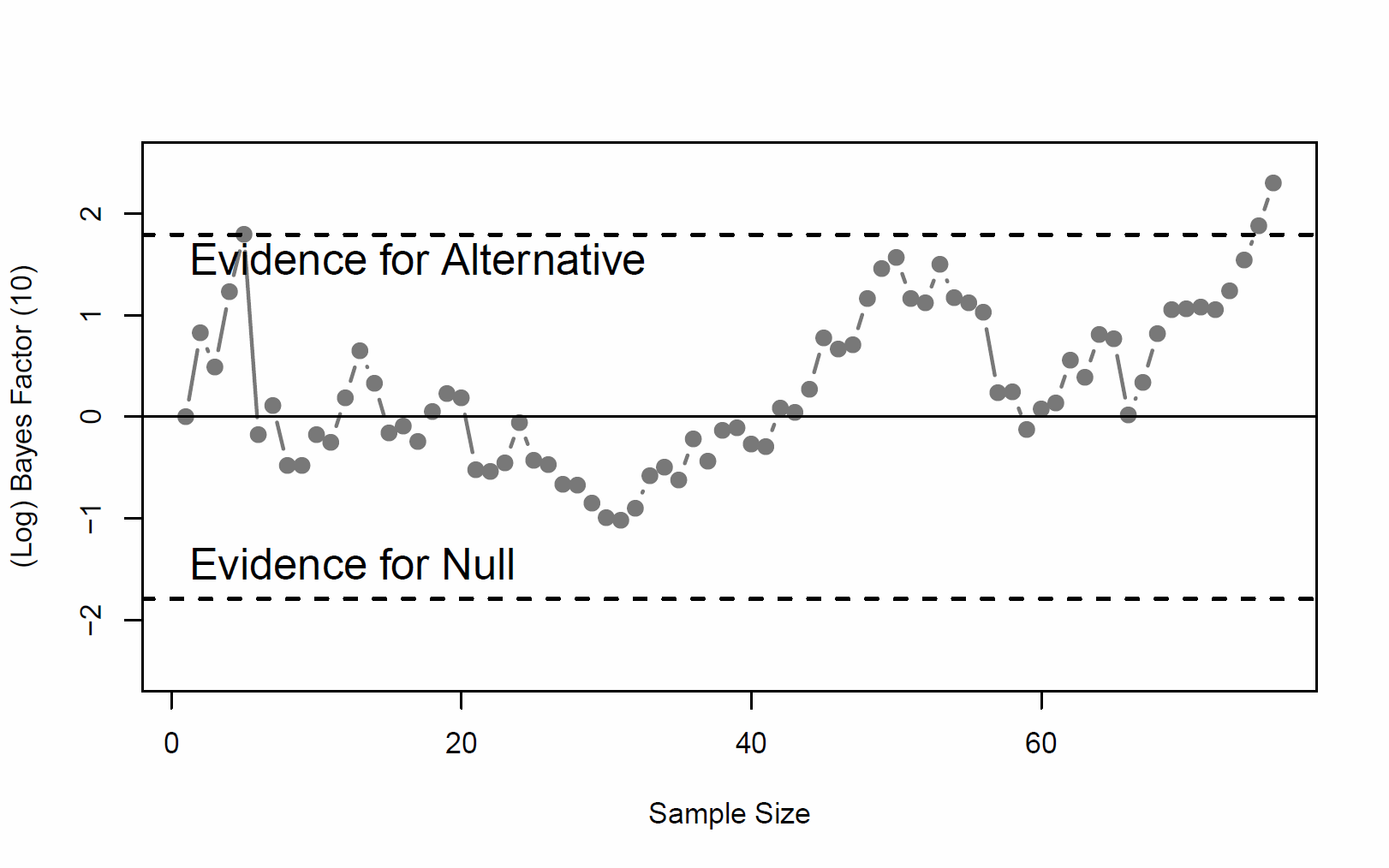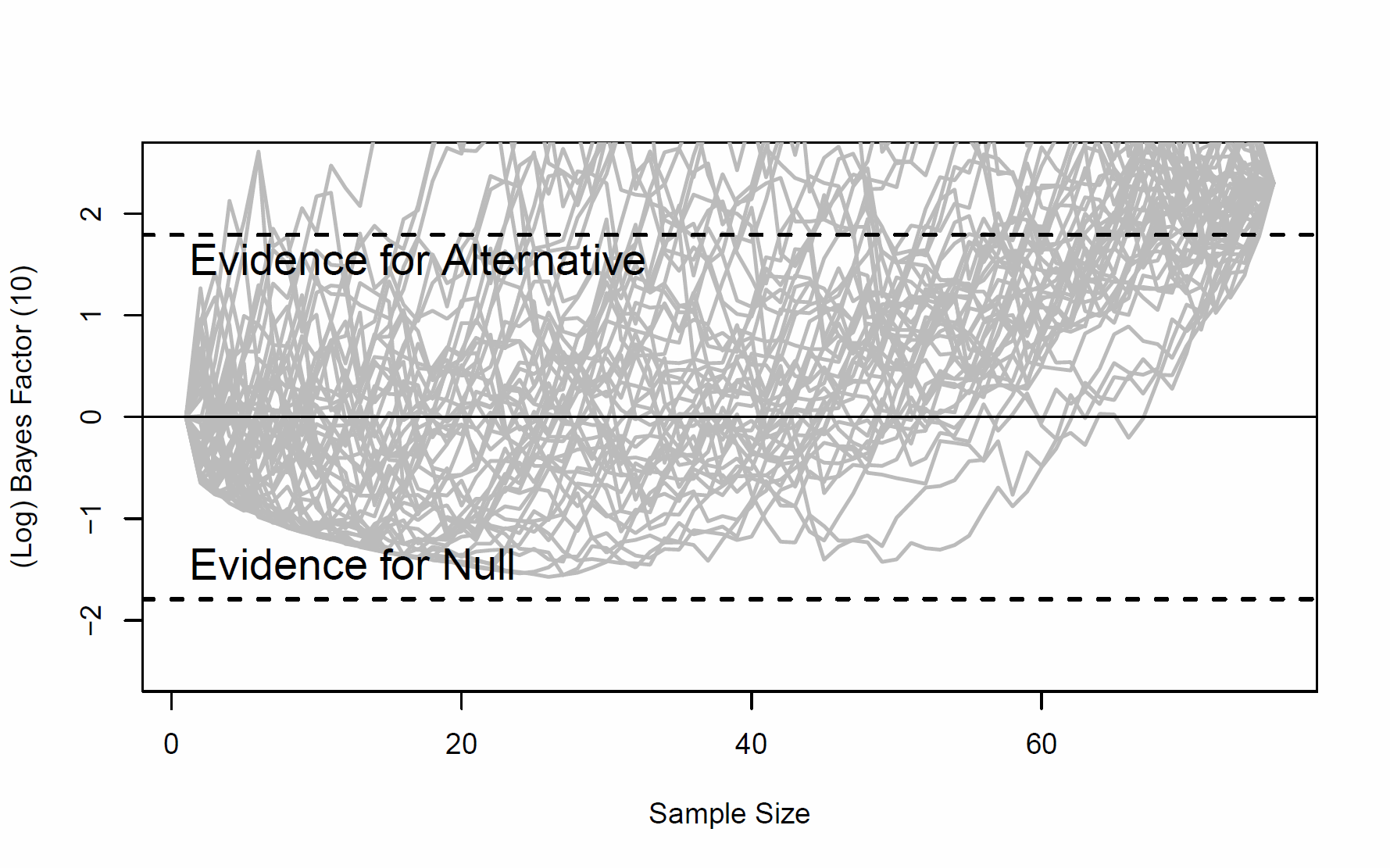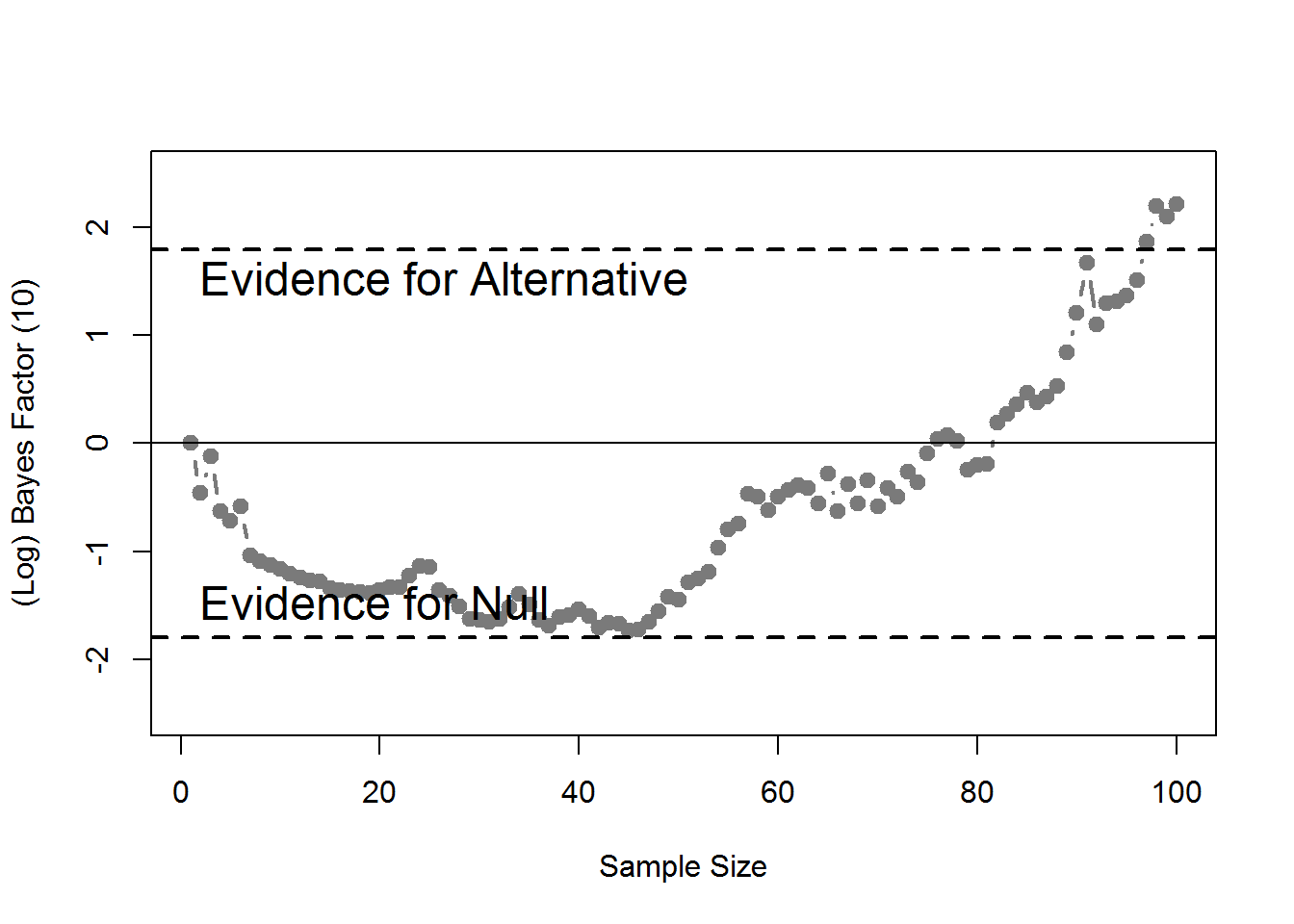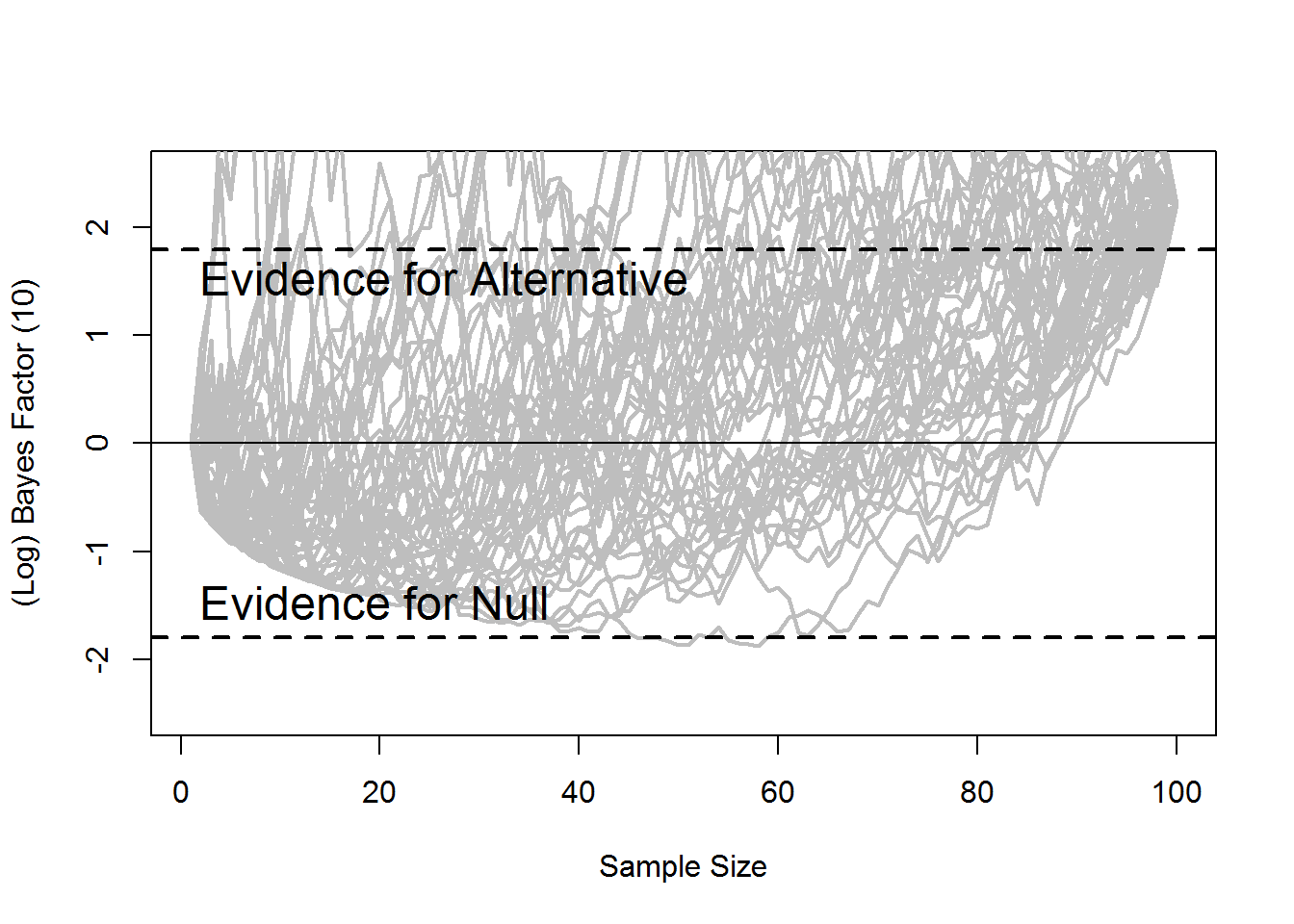One advantage of using Bayes factors (or any other form of Bayesian analysis) is the ability to engage in optional stopping. That is, one can collect some data, perform the critical Bayesian test, and stop data collection once a pre-defined criterion has been obtained (e.g., until “strong” evidence has been found in support of one hypothesis over another). If the criterion has not been met, one can resume data collection until it has.

Optional stopping is problematic when using p-values, but is “No problem for Bayesians!” (Rouder, 2014; see also this blog post from Kruschke). A recent paper in press at Psychological Methods (Shoenbrodt et al., in press) shows you how to use optional stopping with Bayes factors—so-called “sequential Bayes factors” (SBF).

The figure below a recent set of data from my lab where we used SBF for optional stopping. Following the advice of the SBF paper, we set our stopping criteria as N > 20 and BF10 > 6 (evidence in favour of the alternative) or BF10 < 1/6 (evidence in favour of the null); these criteria are shown as horizontal dashed lines. The circles represent the progression of the Bayes factor (shown in log scale) as the sample size increased. We stopped at 76 participants, with “strong” evidence supporting the alternative hypothesis.One thing troubled me when I was writing these results up: I could well imagine a skeptical reviewer noticing that the Bayes factor seemed to be in support of the null between sample sizes of 30 and 40, although the criterion for stopping was not reached. A reviewer might wonder, therefore, whether had some of the later participants who showed no effect (see e.g., participants 54–59) been recruited earlier, would we have stopped data collection and found evidence in favour of the null? Put another way, how robust was our final result to the order in which our sample were recruited to the experiment?

## Assessing the Effect of Recruitment Order

I was not aware of any formal way to assess this question, so for the purposes of the paper I was writing I knocked up a quick simulation. I randomised the recruitment order of our sample, and plotted the sequential Bayes factor for this “new” recruitment order. I performed this 50 times, plotting the trajectory of the SBF.

I reasoned that if our conclusion was robust against recruitment order, the stopping rule in favour of the null should be rarely (if ever) met. The results of this simulation are below. As can be seen, the stopping criterion for the null was never met, suggesting our results were robust to recruitment order.## Recruitment Order Simulation

suppressWarnings(suppressMessages(library(BayesFactor)))
### declare the function that calculates the sequential Bayes factor
bfProg <- function(data, scale = 0.707){

# blank matrix to store data in
bfProg <- matrix(0, ncol = 2, nrow = length(data))
colnames(bfProg) <- c("N", "BF")

for(i in 1:length(data)){

if(i == 1){
bfProg[i, 1] <- 1
bfProg[i, 2] <- 0
}

if(i > 1){
tempData <- data[1:i]
bfProg[i, 1] <- i
bf <- ttestBF(x = tempData, rscale = scale)
bfProg[i, 2] <- exp(bf@bayesFactor\$bf)
bfProg[i, 2] <- log(bfProg[i, 2])
}
}
return(bfProg)
}

Then, I became curious: How robust are SBFs to recruitment order when we know what the true effect is? I simulated a set of 100 participants for an effect known to be small (d = 0.3), and plotted the SBF as “participants” were recruited. I tried different random seeds until I found a pattern I was interested in: I wanted a set of data where the SBF comes very close to the stopping rule in favour of the null, even though we know the alternative is true. It took me a few tries, but eventually I got the plot below.

# set random seed so that user can recreate plot
set.seed(20)

# how many subjects in the study?
nSubjects <- 100

# what is the effect size?
effSize <- 0.3

# get the data for
data <- rnorm(nSubjects, effSize)

# get the sequential Bayes factor for this data
bf <- bfProg(data)

# now plot it
plot(bf[, 1], bf[, 2], type = "b", xlab = "Sample Size",
ylab = "(Log) Bayes Factor (10)", ylim = c(-2.5, 2.5), pch = 19,
col = "gray48", lwd = 2)

abline(h = 0, lwd = 1)
abline(h = log(6), col = "black", lty = 2, lwd = 2)
abline(h = log(1/6), col = "black", lty = 2, lwd = 2)
text(0, log(3) + 0.4, labels = "Evidence for Alternative",
cex = 1.5, col = "black", pos = 4)
text(0, log(1/3) - 0.4, labels = "Evidence for Null",
cex = 1.5, col = "black", pos = 4)Note the dip toward the null criterion is even more extreme than in my experiment. Thus, this set of data seemed perfect to test my method for assessing the robustness of the final conclusion (“strong evidence for alternative”) against recruitment order. I followed the same procedure as before, simulating 50 random recruitment orders, and plotted the results below.

### simulation showing recruitment order does not change stopping rule

# set random seed so user can re-produce plot
set.seed(42)

# how many "experiments" to simulate?
nSims = 50

# get a matrix to store all of the "experiments" in
orderAnalysis <- matrix(0, nrow = length(data), ncol = nSims)

# fill the matrix with random recruitment orders
for(i in 1:nSims){

# simulate new ordering
orderData <- base::sample(data, size = length(data),
replace = FALSE)
bf <- bfProg(orderData, scale = 0.707)
orderAnalysis[, i] <- bf[, 2]
orderAnalysis[1, i] <- 0
}

# plot the progression of the Bayes Factor of the interaction as more

# plot the first "experiment"
plot(seq(1:length(data)), orderAnalysis[, 1], type = "l",
xlab = "Sample Size", ylab = "(Log) Bayes Factor (10)",
ylim = c(-2.5, 2.5), pch = 19, col = "gray", lwd = 2)

# now do the rest
for(i in 2:nSims){
lines(seq(1:length(data)), orderAnalysis[, i], type = "l", col = "gray",
lwd = 2)
}

cex = 1.5, col = "black", pos = 4)